# 开发小技巧系列 - 从BigDecimal的divide的异常说起

``//省略业务的逻辑 ...//得到订单金额BigDecimal orderAmount = new BigDecimal(2312.23);//得到成本BigDecimal cost = new BigDecimal(984.23);//得到客户数BigDecimal customerCount = new BigDecimal(35);//得到客单价BigDecimal pricePerCustomer = orderAmount.divide(customerCount);log.info("客单价：{}", pricePerCustomer);//得到毛利率BigDecimal profit = (orderAmount.subtract(cost)).divide(cost);log.info("毛利率：{}", profit);//省去其他逻辑...``

``11:01:10.027 [main] INFO net.jhelp.demo.BigDecimalTest - 客单价：66.06371428571428623399697244167327880859375java.lang.ArithmeticException: Non-terminating decimal expansion; no exact representable decimal result.	at java.math.BigDecimal.divide(BigDecimal.java:1690)``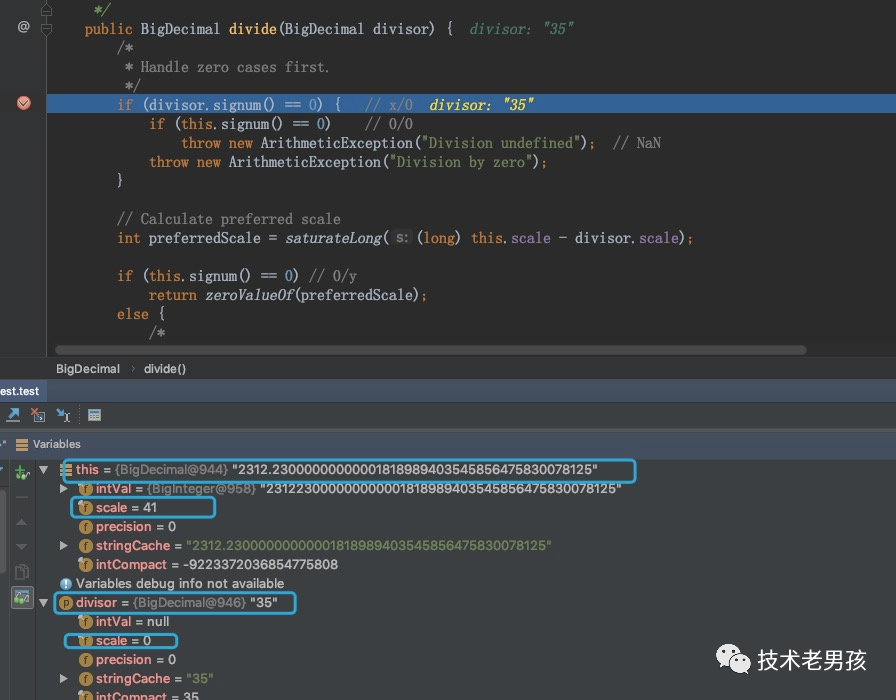new BigDecimal(2312.23)实际上调用的是BigDecimal中的浮点数构造方法：

``````public BigDecimal(Double value){
.....
this.intVal = intVal;
this.intCompact = compactVal;
this.scale = scale;
this.precision = prec;
}``````

new BigDecimal(35)调用的是BigDecimal中的整数构造方法：

``````public BigDecimal(int val) {
this.intCompact = val;
this.scale = 0;
this.intVal = null;
}``````

再继续往下走，来看看preferredScale预期的小数位精度）是多少。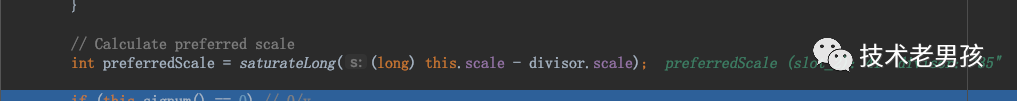这时的preferredScale=41, 继续往下看。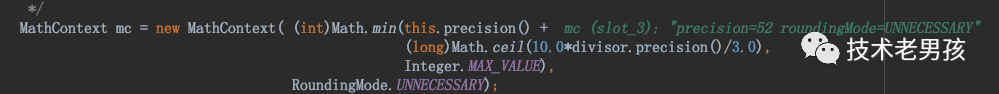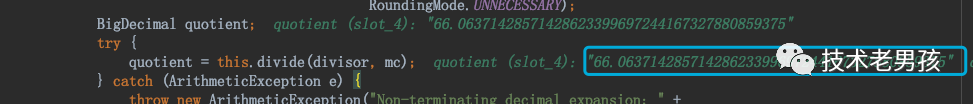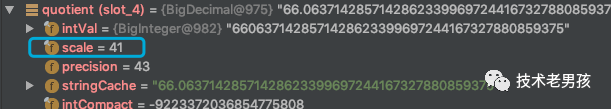从上图中看到，BigDecimal在计算2个除数的商时，如果被除数是整数，那商的精度由除数的精度决定（从计算preferredScale可以看出）。另外，还生成了一个MathContext的对象，默认进位规则是：RoundingMode.UNNECESSARY

这里面有一个重要的分支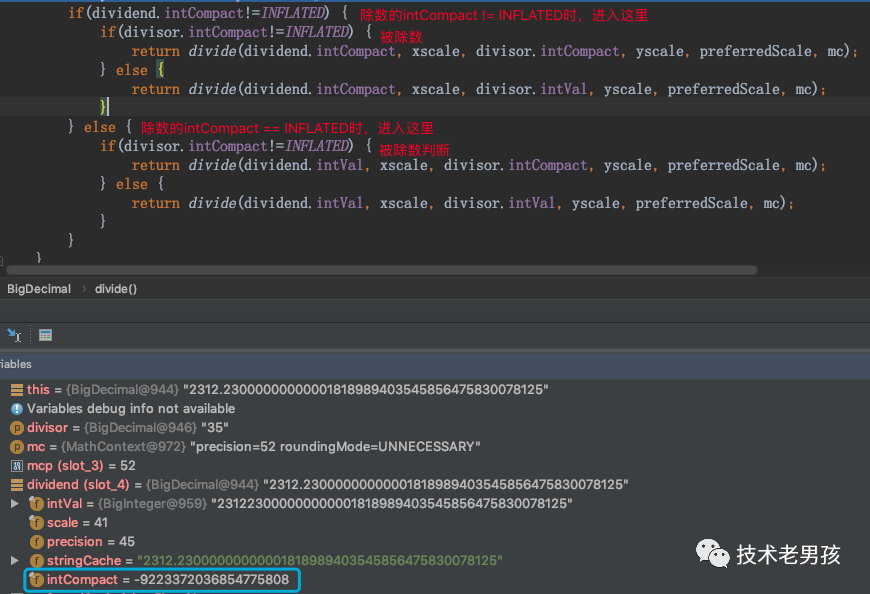当BigDecimal是一个浮点类型的数时，它的intCompact是和INFLATED的值相同的。

方法的调用流程是这样：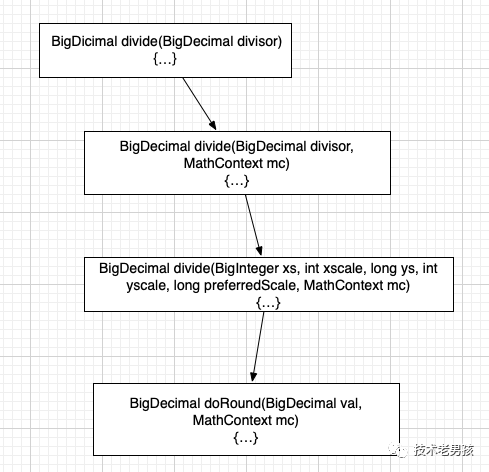最终返回一个跟“除数”一样精度的“商”值。

来看第二个divide的跟踪过程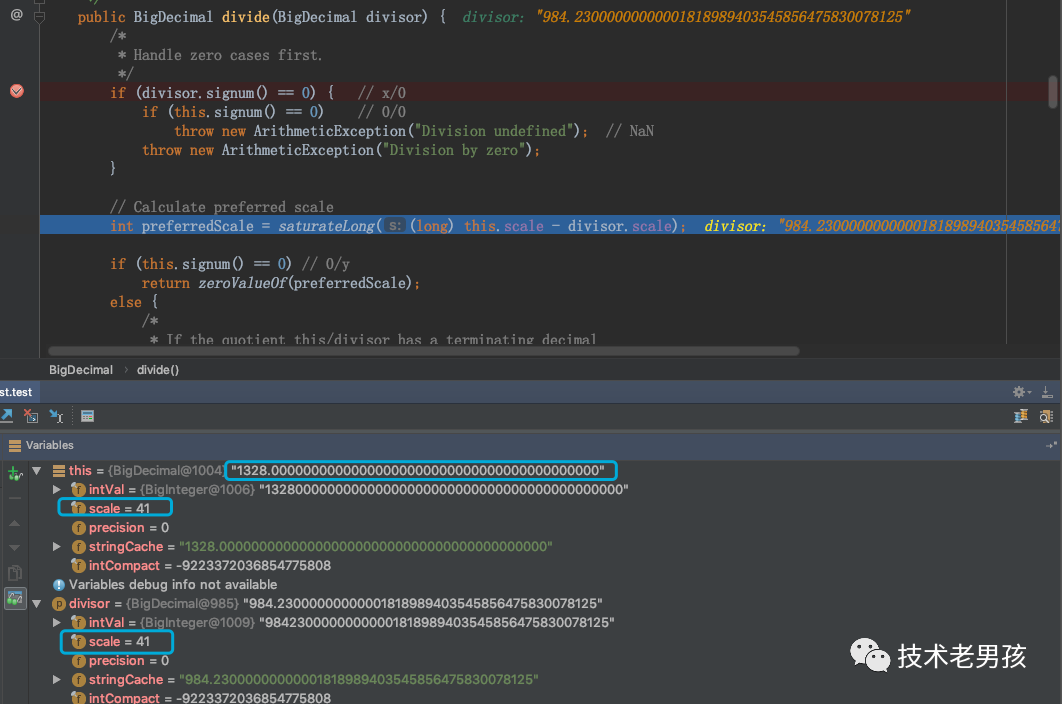第二个divide的除数，被除数的scale=41, intCompact的值相同（-9223372036854775808==INFLATED）preferredScale=0，而这时的MathContext 与前一个有很大的不同，它的precision=192.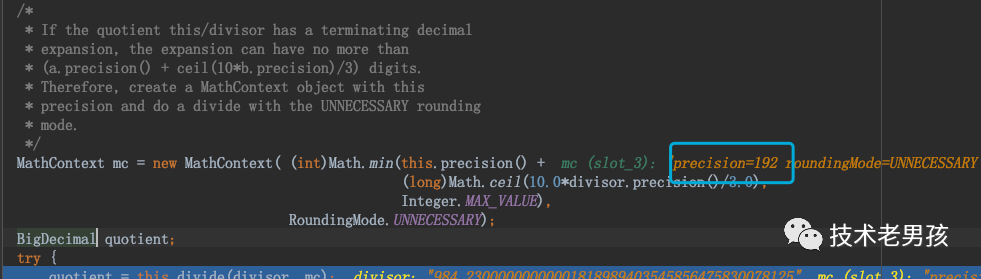``````this.precision() = 45
(long)Math.ceil(10.0*divisor.precision()/3.0 = 147``````

而在这个逻辑分支时，2个浮点相除的，走的是下面的方法，和第一个计算时走的分支是不同的，第一个计算走的是上面的方法。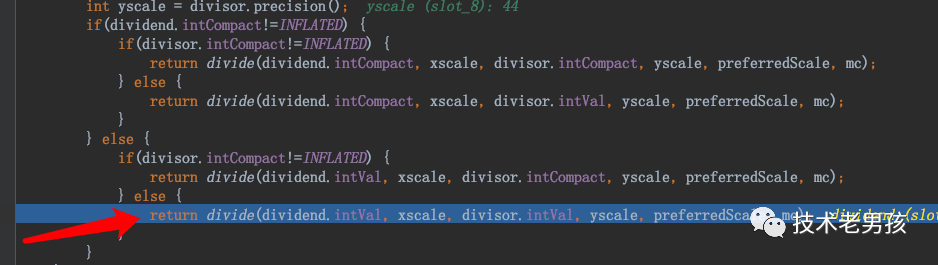来看看它的方法调用流程：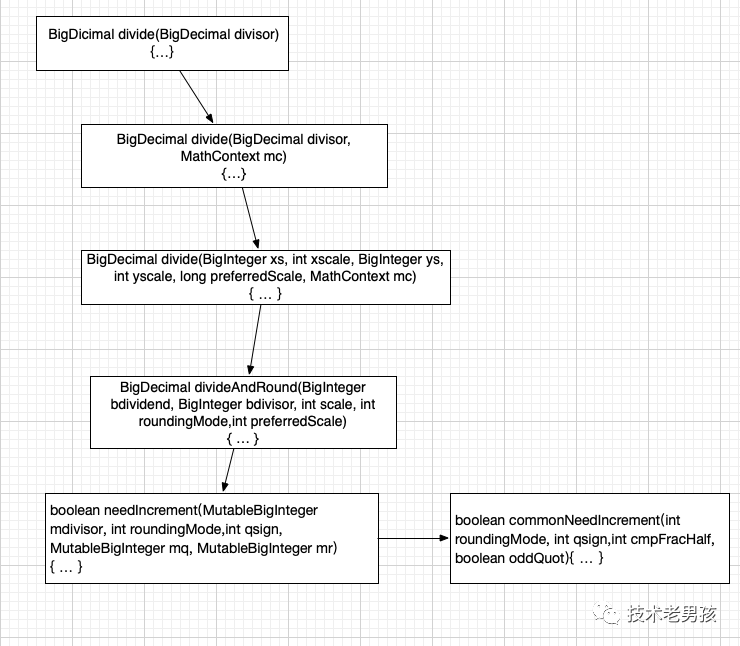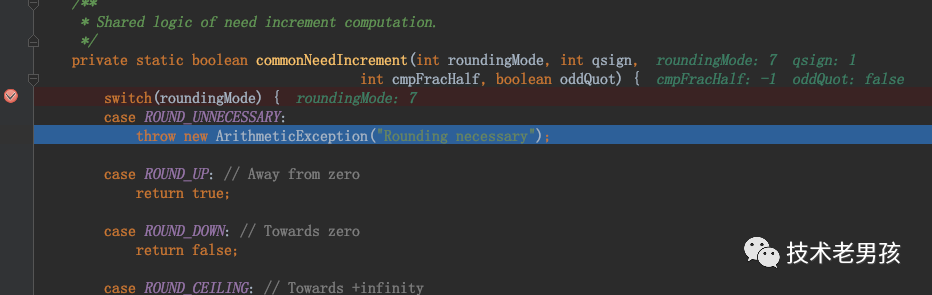``````    /**
* Shared logic of need increment computation
.     */
private static boolean commonNeedIncrement(int roundingMode, int qsign,                                     int cmpFracHalf, boolean oddQuot) {

switch(roundingMode) {
case ROUND_UNNECESSARY:
throw new ArithmeticException("Rounding necessary");        case ROUND_UP: // Away from zero
return true;        case ROUND_DOWN: // Towards zero
return false;
//略...
}``````

跟踪到这里，是不是清晰很多了，这里的roundingMode就是在调用divide的方法中获取的，也是是前面的MathContext对象生成时，默认设置的RoundingMode.UNNECESSARY，从这个方法的调用过程中，可以看出divide(BigDecimal divisor）方法不适合用于2个数都是“浮点数“的场景的相除。（题外话，这里抛出的异常，与外面见到的异常是不一样的，有点坑，为什么这个方法不标志一下@Deprecated，让别人不要掉坑里。

总结

上面根据异常的信息，对BigDecimal的divide（除法）的源代码分析与跟踪，分析出现问题的原因，在开发的过程中，不要使用divide(BigDecimal divisor）这方法，以免掉到坑里，尽理使用divide(BigDecimal divisor, int scale, int roundingMode)这种，指定精度，和“舍入规则”。

<end>

文字点滴，希望你能收获点滴，觉得不错的可以点个赞。

开发小技巧系列文章：

7. 开发小技巧系列 - 如何避免NPE，去掉if...else（四）

本文链接：https://www.jhelp.net/p/th9lFzu0fgk7UOlo (转载请保留)。
关注下面的标签，发现更多相似文章